# Bash Script – Arithmetic Operators

• Last Updated : 04 Jan, 2022

In this article, we will see arithmetic operators in bash script. Arithmetic operators is used to perform arithmetic operations.

Bash script supports 11 arithmetic operators. All the operators with their uses is given below:

Let’s see the examples for the uses of arithmetic operators:

Code:

```Sum=\$((10+3))
echo "Sum = \$Sum"  ```

Output: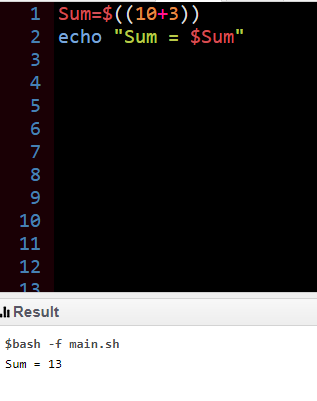### Subtraction

Code:

```Difference=\$((10-3))
echo "Difference = \$Difference"  ```

Output: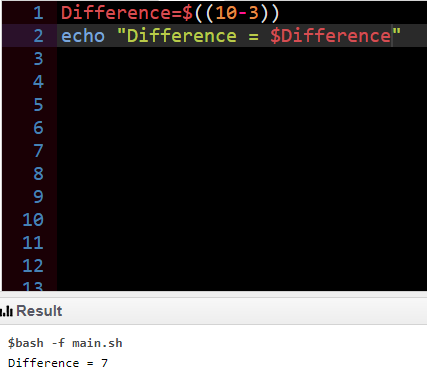### Multiplication

Code:

```Product=\$((10*3))
echo "Product = \$Product"  ```

Output:

[Image_Link]https://media.geeksforgeeks.org/wp-content/upl

### Division

Code:

```Division=\$((10/3))
echo "Division = \$Division"  ```

Output: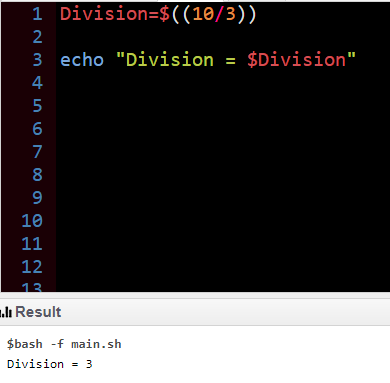### Modulo

Code:

```Modulo=\$((10%3))
echo "Modulo = \$Modulo"  ```

Output: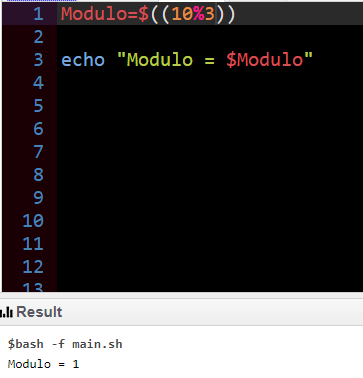### Exponentiation

Code:

```Exponent=\$((10**2))
echo "Exponent = \$Exponent"  ```

Output: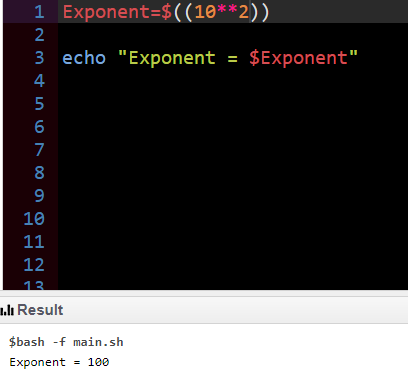Example to show use of a

he operators in a single code

Code:

```x=10
y=20
echo "x=10, y=5"
echo "Addition of x and y"
echo \$(( \$x + \$y ))
echo "Subtraction of x and y"
echo \$(( \$x - \$y ))
echo "Multiplication of x and y"
echo \$(( \$x * \$y ))
echo "Division of x by y"
echo \$(( \$x / \$y ))
echo "Exponentiation of x,y"
echo \$(( \$x ** \$y ))
echo "Modular Division of x,y"
echo \$(( \$x % \$y ))
echo "Incrementing x by 10, then x= "
(( x += 10 ))
echo \$x
echo "Decrementing x by 15, then x= "
(( x -= 15 ))
echo \$x
echo "Multiply of x by 2, then x="
(( x *= 2 ))
echo \$x
echo "Dividing x by 5, x= "
(( x /= 5 ))
echo \$x
echo "Remainder of Dividing x by 5, x="
(( x %= 5 ))
echo \$x  ```

Output: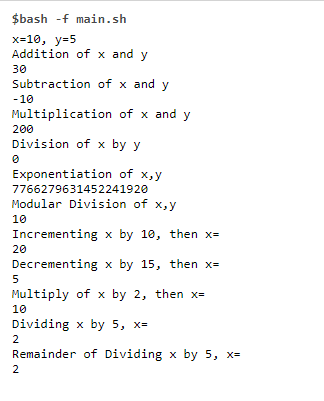## Different ways to compute Arithmetic Operations in Bash

There are some of the different ways to perform Arithmetic Operations.

### 1. Double Parenthesis

This could be used for arithmetic expansion. Let’s see an example to see the use of double quotes.

Code:

```#!/bin/bash

first=10
second=3

echo \$(( first + second ))   # addition
echo \$(( \$first + \$second )) # this is also valid

echo \$(( first - second ))   # subtraction
echo \$(( \$first - \$second )) # this is also valid
```

Output:

```13
13
7
7```

### 2. Using let command

let command is used to perform arithmetic operations.

Example

code:

```#!/bin/bash

x=10
y=3

let "z = \$(( x * y ))"  # multiplication
echo \$z
let z=\$((x*y))
echo \$z

let "z = \$(( x / y ))"  # division
echo \$z
let z=\$((x/y))
echo \$z```

Output:

```30
30
3
3```

### 3. expr command with backticks

Arithmetic expansion could be done using backticks and expr.

Code:

```a=10
b=3

# there must be spaces before/after the operator

sum=`expr \$a + \$b`
echo \$sum

sub=`expr \$a - \$b`
echo \$sub

mul=`expr \$a \* \$b`
echo \$mul

div=`expr \$a / \$b`
echo \$div```

Output:

```13
7
30
3```
My Personal Notes arrow_drop_up